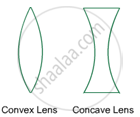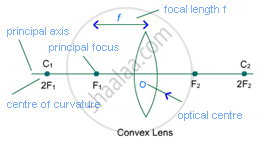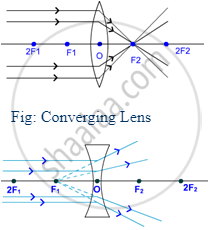Karnataka Secondary Education Examination BoardKarnataka SSLC
Share

# Refraction of Light - Introduction

#### notes

SPHERICAL LENS

Lens is an optical device which converges or diverges the rays of light before transmitting. A lens has similar shape to lentils and genus of lentil is called Lens, thus a lens got its name after the shape and name of genus of lentils. A lens is made by combining at least one part of sphere made of transparent material, generally glass.

Spherical Lens: Most of the lenses are made by the combination of parts of transparent sphere. Concave and Convex lens are most commonly use spherical lens.

Convex lens is the most commonly used lens in our day to day life.

Convex lens:

A lens having two spherical surface bulging outwards is called Convex Lens. It is also known as biconvex lens because of two spherical surface bulging outwards.### Concave lens:

A lens having two spherical surface bulging inwards is called Concave Lens. It is also known as biconcave lens because of two spherical surface bulging inwards.

#### Important terms for spherical lens:Centre of curvature: The centre of sphere of part of which a lens is formed is called the centre of curvature of the lens. Since concave and convex lenses are formed by the combination of two parts of spheres, therefore they have two centres of curvature.

One centre of curvature is usually denoted by C1 and second is denoted by C2.

Focus: Point at which parallel rays of light converge in a concave lens and parallel rays of light diverge from the point is called Focus or Principal Focus of the lens.Similar to centres of curvature; convex and concave lenses have two Foci. These are represented as F1 and F2.

Principal Axis: Imaginary line that passes through the centres of curvature of a lens is called Principal Focus.

Optical centre: The central point of a lens is called its Optical Centre. A ray passes through optical centre of a lens without any deviation.

Radius of curvature: The distance between optical centre and centre of curvature is called the radius of curvature, which is generally denoted by R.

Focal Length: The distance between optical centre and principal focus is called focal length of a lens. Focal length of a lens is half of the radius of curvature.

2F = R

Or, F = "R"/2

This is the cause that the centre of curvature is generally denoted by 2F for a lens instead of C.

### Shaalaa.com

Light Reflection and Refraction part 12 (Numericals) [00:14:01]
S
series 1
0%

S